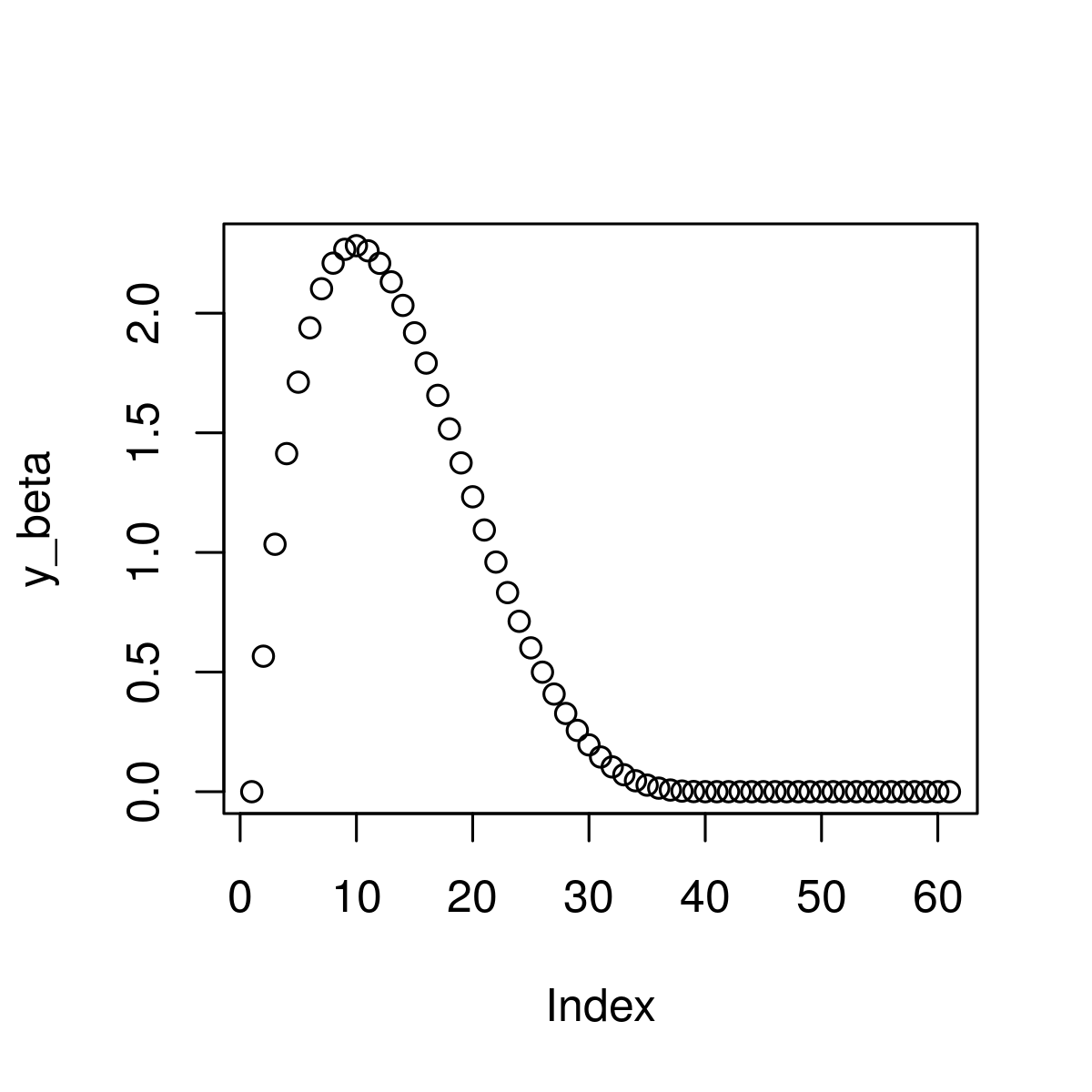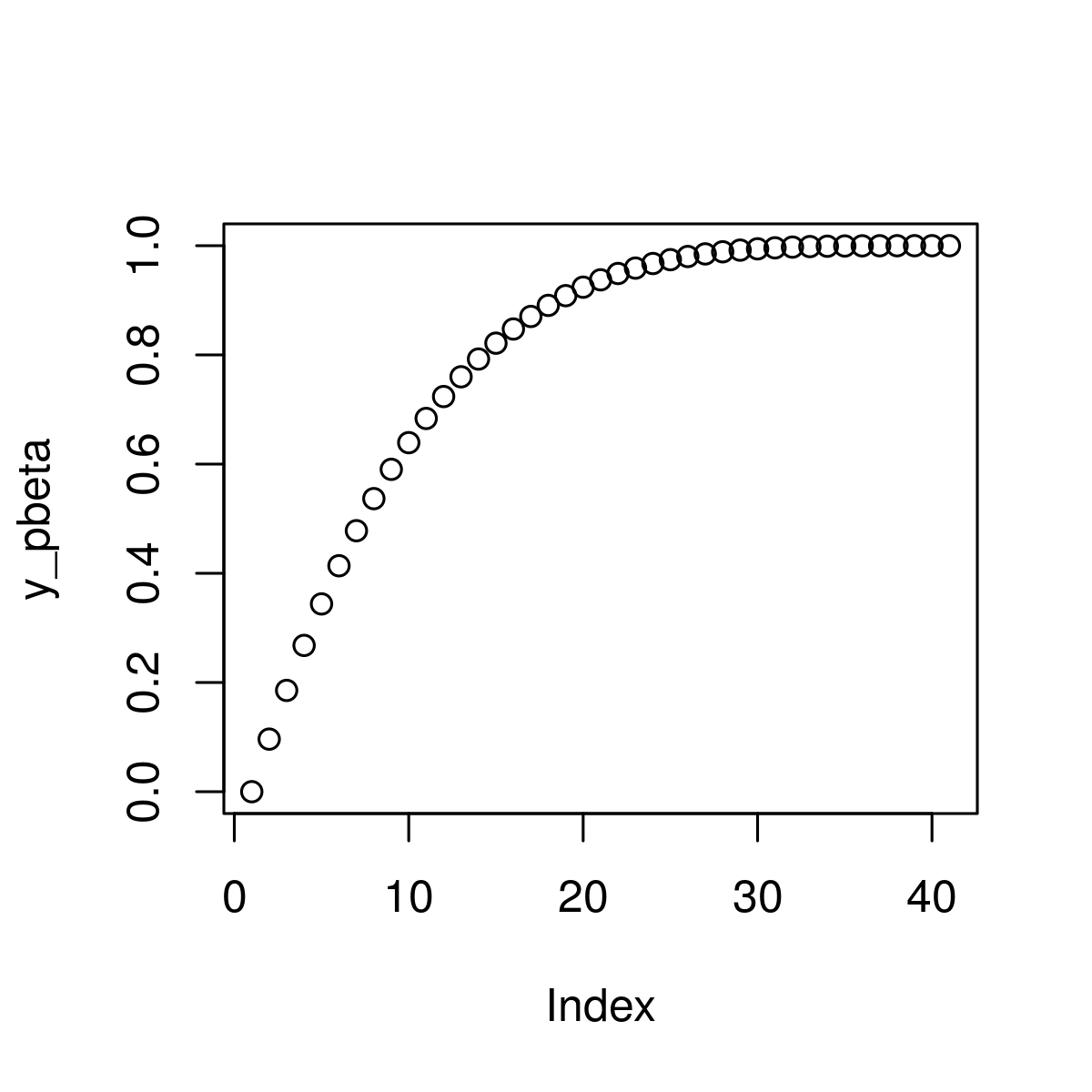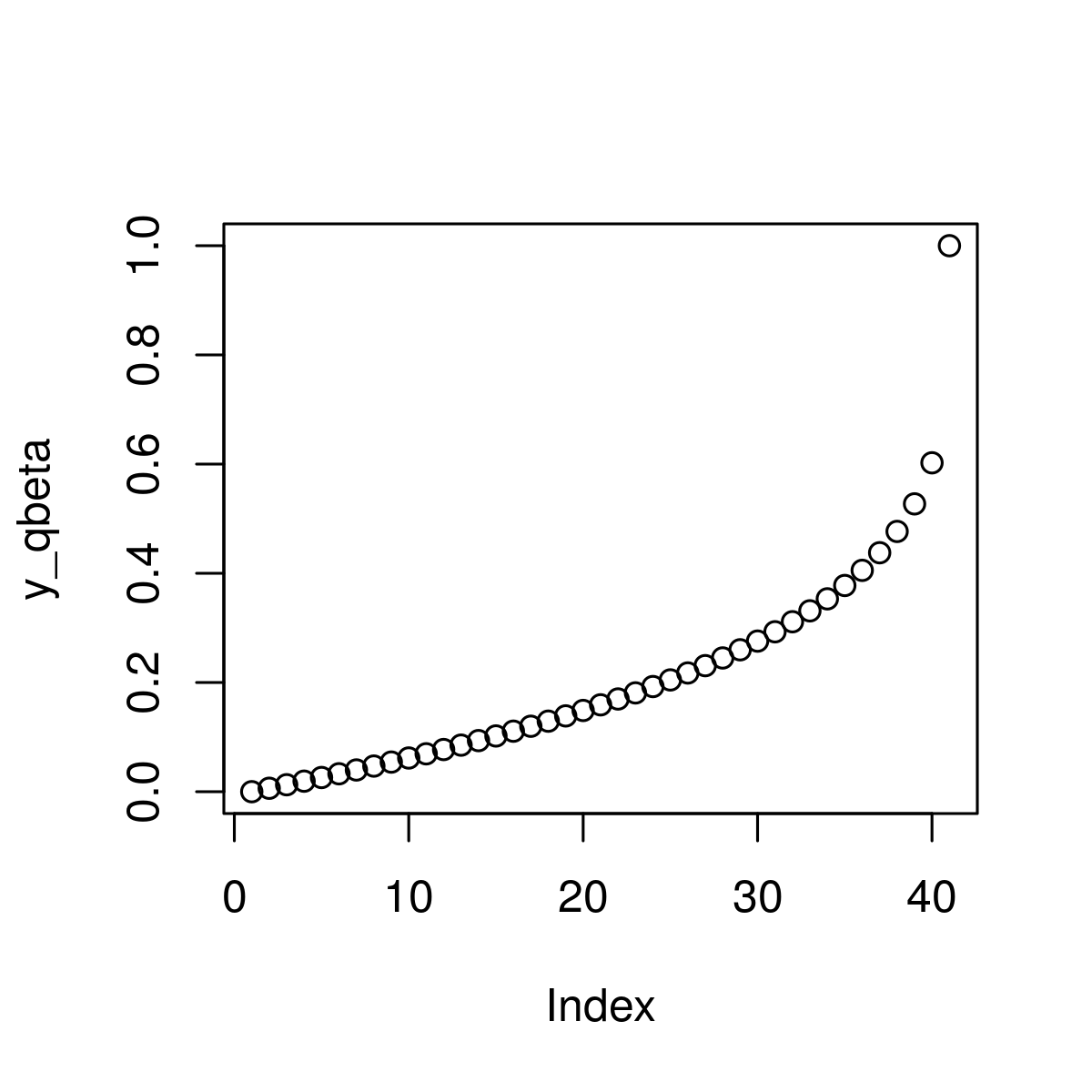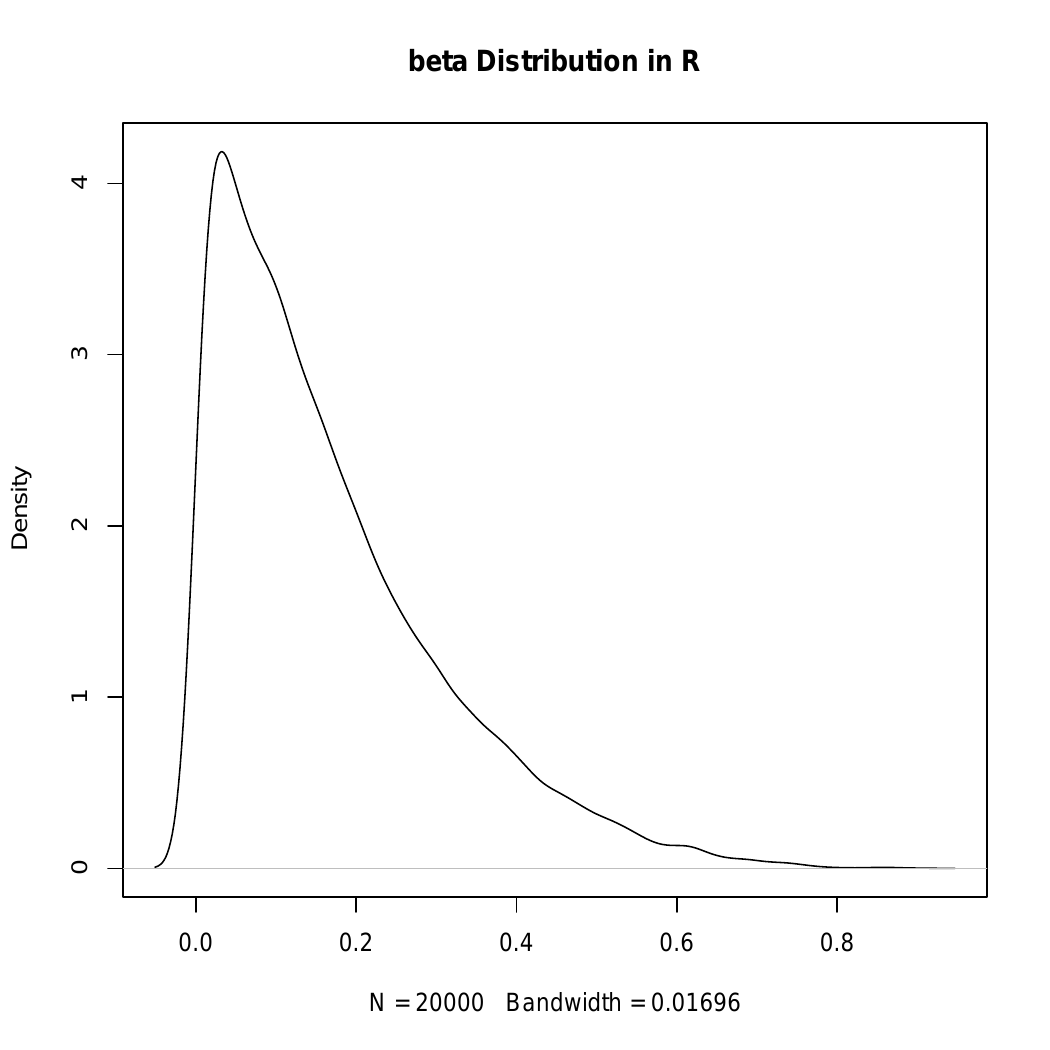# Compute Beta Distribution in R Programming – dbeta(), pbeta(), qbeta(), and rbeta() Functions

Beta Distribution in R Language is defined as property which represents the possible values of probability. This article is an illustration of dbeta, pbeta, qbeta, and rbeta functions of Beta Distribution.

#### dbeta() Function

It is defined as Beta Density function and is used to create beta density value corresponding to the vector of quantiles.

Syntax:
dbeta(vec, shape1, shape2)

Parameter:
vec: Vector to be used
shape1, shape2: beta density of input values

Returns: beta density values for a vector of quantiles

Example :

 `# R program to illustrate the use of  ` `# dbeta() function ` ` `  `# Creating a vector ` `x_beta <- ``seq``(0, 1.5, by = 0.025 )   ` ` `  `# Apply beta function           ` `y_beta <- ``dbeta``(x_beta, shape1 = 2, shape2 = 4.5)   ` ` `  `# Plot beta values ` `plot``(y_beta)                                        `

Output:#### pbeta() Function

It is used to create cumulative distribution function of the beta distribution.

Syntax:
pbeta(vec, shape1, shape2)

Parameter:
vec: Vector to be used
shape1, shape2: beta density of input values

Example:

 `# Specify x-values for pbeta function ` `x_pbeta <- ``seq``(0, 1, by = 0.025)       ` ` `  `# Apply pbeta() function ` `y_pbeta <- ``pbeta``(x_pbeta, shape1 = 1, shape2 = 4)   ` ` `  `# Plot pbeta values ` `plot``(y_pbeta) `

Output:#### qbeta() Function

It is known as beta quantile function and used t return quantile values of the function.

Syntax:
qbeta(vec, shape1, shape2)

Parameters:
vec: Vector to be used
shape1, shape2: beta density of input values

Example:

 `   `  `# Specify x-values for qbeta() function ` `x_qbeta <- ``seq``(0, 1, by = 0.025) ` ` `  `# Apply qbeta() function ` `y_qbeta <- ``qbeta``(x_qbeta, shape1 = 1, shape2 = 4)   ` ` `  `# Plot qbeta() values ` `plot``(y_qbeta)  `

Output:#### rbeta() Function

It is defined as a random number generator that is used to set seed and specify sample size.

Syntax:
rbeta(N, shape1, shape2 )

Parameters:
vec: Vector to be used
shape1, shape2: beta density of input values

Example:

 `# Set seed for reproducibility ` `set.seed``(13579) ` ` `  `# Specify sample size ` `N <- 10000   ` ` `  `# Draw N beta distributed values ` `y_rbeta <- ``rbeta``(N, shape1 = 1, shape2 = 5)    ` `y_rbeta ` ` `  `# Plot of randomly drawn beta density ` `plot``(``density``(y_rbeta),  ` `     ``main = ``"beta Distribution in R"``) `

Output:My Personal Notes arrow_drop_upCheck out this Author's contributed articles.

If you like GeeksforGeeks and would like to contribute, you can also write an article using contribute.geeksforgeeks.org or mail your article to contribute@geeksforgeeks.org. See your article appearing on the GeeksforGeeks main page and help other Geeks.

Please Improve this article if you find anything incorrect by clicking on the "Improve Article" button below.

Article Tags :

Be the First to upvote.

Please write to us at contribute@geeksforgeeks.org to report any issue with the above content.